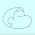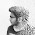I2C Verilog Code Explanation II

In my previous post, I explained working of I2C Verilog code. Same is continued here.

else if(left_bits == 10)begin
if(sda == 0)begin
left_bits <= 1;
direction <= 1;
temp <= temp_reserved;
end
else begin
direction <= 1;
alpha <= 0;
left_bits <= left_bits + 1;
end
end

When the ACK/NACK is received at 9 then at 10 it is compared with 1 and 0. If the acknowledgment received is 0 then left_bits is reset to its initial value that is 1. The direction is again set to 1 to make the Master ready to send data to Slave. The register TEMP which is now XXXXXXX gets renewed or say reset with a copy that we stored earlier i.e. in TEMP_RESERVED. If the received acknowledgment is 1 then direction will be changed to 1 because now the Master will have to send the address of register which stores data in the Slave. Setting ALPHA = 0 is not necessary here though. LEFT_BITS is again incremented.

else if(left_bits >=11 && left_bits <=17)begin
alpha <= register;
register <= register<<1;
left_bits <= left_bits + 1;
end

From 11 to 17 i.e. 7 counts the Master will send the address of the register of the Slave from where data has to be retrieved. The process is same as I explained earlier to send Slave address hence I am not gonna repeat the same.

else if (left_bits >= 18 && left_bits <= 28)begin
direction <=0;
left_bits <= left_bits + 1;
end

From 18 to 28 the slave will send an ACK bit to tell the master that if the register address is matched or not. This happens on 19th cycle. However, there is a clock cycle delay here in Slave at this position. The SDA line is continuous from Master to Slave. Since we cannot do any operation on wires, therefore, we have to store data from wire to a register in the Slave in order to use it. However, storing this data results in an extra clock cycle, since values get updated after the clock cycle and not along with clock cycle for a non-blocking assignment (<=). For blocking assignment (=) the task is done first having the blocking assignment and the rest of the tasks are completed.

Example
initial begin
a = 0;
c = 0;
end
always @(posedge clk)begin
a <= a + 1;
a <= a + 2;
c <= c + 1;
a <= a + 3;
c <= c + 2;
end

After executing the above command if you are thinking that the result will be a = 6 and c = 3, then you are wrong !!.
The right answer is a = 3 and c = 2;

However, if you code like this snippet below using blocking assignments
always @(posedge clk)begin
a = a + 1;
a = a + 2;
c = c + 1;
a = a + 3;
c = c + 2;
end
The output will be a = 6 and c = 2;

Whenever we use non-blocking assignments each line of code does not depend on the previous line. They will occur simultaneously with the past data. Data is changed after the clock cycle ends and not immediately. In case of blocking assignment, the first line will be given first priority. After it's execution, the second line is executed. Data is changed immediately.
Thus
a <= a + 1; mean a = 0 + 1 = 1
a <= a + 2 means a = 0 + 2 = 2 . It won't depend on the previous calculation.
a <= a + 3 means a = 0 + 3 = 3   It won't depend on the previous calculation
At the end of clock cycle a = 3

However,
a = a + 1 means a = 0 + 1 = 1. This will be executed immediately.
a = a + 2 means a = 1 + 2 = 3 This will be executed after the previous execution
a = a + 3 means a = 3 + 3 = 6.

Non-blocking assignments are used only when sequential conditions are present i.e. flip-flops. Blocking assignments are used for combinational blocks. I hope it is clear the difference between blocking and non-blocking assignments.

To manage this bit delay in slave I had to use a blocking assignment. The Slave sends the 8-bit data stored in its register to Master by setting direction = 0 on the SDA line.

else if(left_bits == 29)begin
direction <= 1;
alpha <= 1;
left_bits <= left_bits + 1;
end

For the 29th count, the Master will set the direction 1 and the Slave will set the direction as 0 as it is the turn of Master to send the acknowledgment bit now informing the Slave that it has received the 8-bit data. The acknowledgment is sent by assigning ALPHA = 1 i.e. SDA is pulled high.

else if(left_bits == 30)begin
alpha <= 0;
left_bits <= left_bits + 1;
end
else if(left_bits == 31)begin
#2 alpha <= 1;
left_bits <= left_bits + 1;
end
else if(left_bits == 32)
a <= 0;
end

At the count 30 ALPHA is set to 0 as ACK is only of 1 bit. For the count 31 ALPHA is set to 1 i.e SDA is pulled high. No negative edge is present afterward here. For the count 32 "a" is set to 0 thus switching SCL line to 1. Since there is not negedge of SDA there SCL won't start clocking again which I have already explained earlier. #2 is the time delay to 2 ns to introduce the STOP condition.

Fig - STOP Condition

Although #1 should be present there as in the above image I mistakenly added an extra clock cycle. You are free to experiment.

I'll explain the SLAVE code later.

So Long

1.This explanation was necessary.
Thanks Buddy

1.Yo But one should try to decode the understanding of code by him/herself

2.HeHeHe Sorry man,
Regards
R John